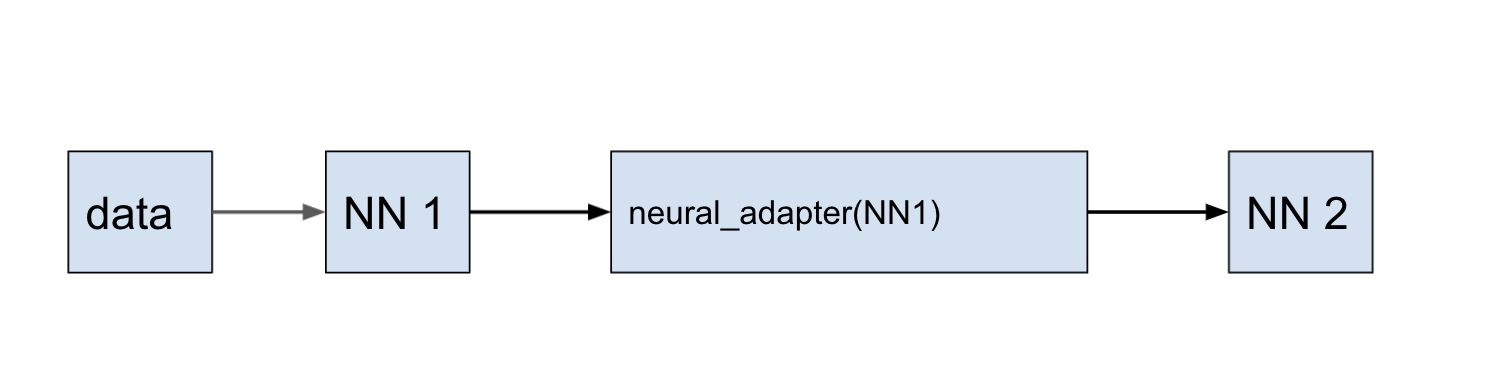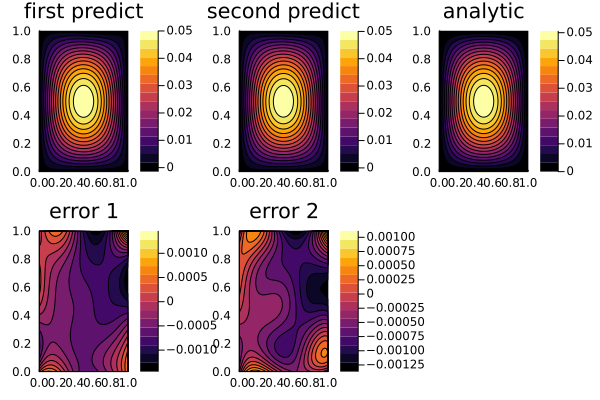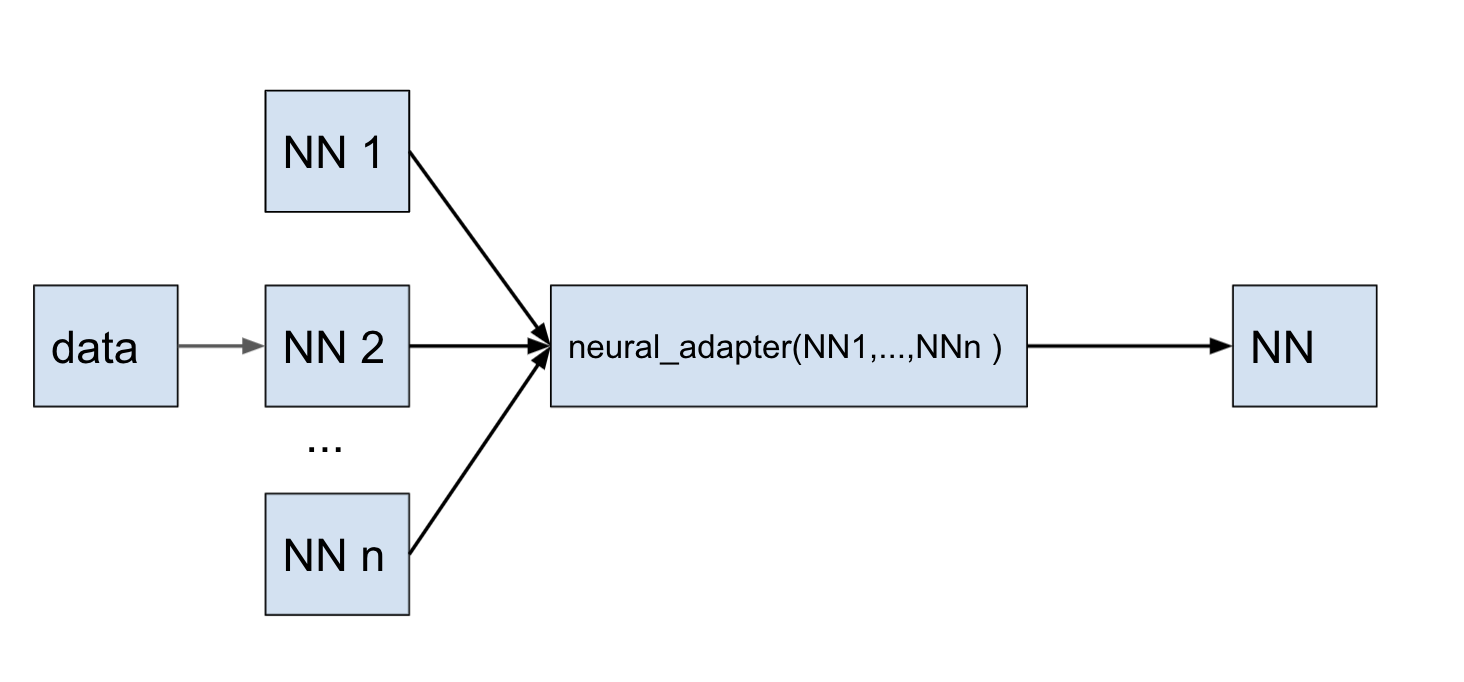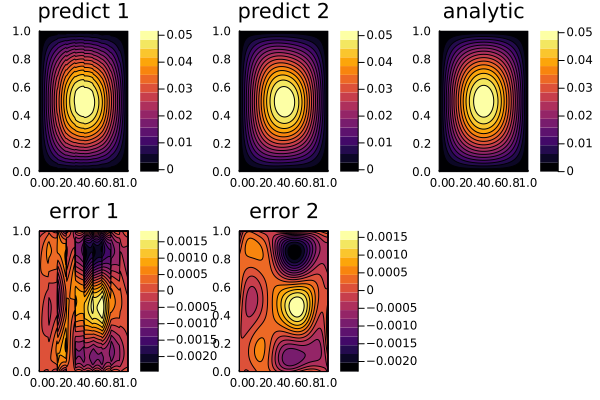# Transfer Learning with Neural Adapter

Transfer learning is a machine learning technique where a model trained on one task is re-purposed on a second related task.

neural_adapter is method that trains a neural network using the results from an already obtained prediction.

This allows reusing the obtained prediction results and pre-training states of the neural network to get a new prediction, or reuse the results of predictions to train a related task (for example, the same task with a different domain). It makes it possible to create more flexible training schemes.

## Retrain the prediction

Using the example of 2D Poisson equation, it is shown how, using method neural_adapter, to retrain the prediction of one neural network to another.using NeuralPDE, Flux, ModelingToolkit, GalacticOptim, Optim, DiffEqFlux, DiffEqBase
import ModelingToolkit: Interval, infimum, supremum

@parameters x y
@variables u(..)
Dxx = Differential(x)^2
Dyy = Differential(y)^2

# 2D PDE
eq  = Dxx(u(x,y)) + Dyy(u(x,y)) ~ -sin(pi*x)*sin(pi*y)

# Initial and boundary conditions
bcs = [u(0,y) ~ 0.0, u(1,y) ~ -sin(pi*1)*sin(pi*y),
u(x,0) ~ 0.0, u(x,1) ~ -sin(pi*x)*sin(pi*1)]
# Space and time domains
domains = [x ∈ Interval(0.0,1.0),
y ∈ Interval(0.0,1.0)]
maxiters =50, batch=100)
inner = 8
af = Flux.tanh
chain1 = Chain(Dense(2,inner,af),
Dense(inner,inner,af),
Dense(inner,1))

initθ = Float64.(DiffEqFlux.initial_params(chain1))
discretization = NeuralPDE.PhysicsInformedNN(chain1,
init_params = initθ)

@named pde_system = PDESystem(eq,bcs,domains,[x,y],[u(x, y)])
prob = NeuralPDE.discretize(pde_system,discretization)
sym_prob = NeuralPDE.symbolic_discretize(pde_system,discretization)

res = GalacticOptim.solve(prob, BFGS();  maxiters=2000)
phi = discretization.phi

inner_ = 12
af = Flux.tanh
chain2 = FastChain(FastDense(2,inner_,af),
FastDense(inner_,inner_,af),
FastDense(inner_,inner_,af),
FastDense(inner_,1))

initθ2 =Float64.(DiffEqFlux.initial_params(chain2))

# the rule by which the training will take place is described here in loss function
function loss(cord,θ)
chain2(cord,θ) .- phi(cord,res.minimizer)
end

strategy = NeuralPDE.GridTraining(0.02)

prob_ = NeuralPDE.neural_adapter(loss, initθ2, pde_system, strategy)
cb = function (p,l)
println("Current loss is: $l") return false end res_ = GalacticOptim.solve(prob_, BFGS();cb=cb, maxiters=1000) parameterless_type_θ = DiffEqBase.parameterless_type(initθ2) phi_ = NeuralPDE.get_phi(chain2,parameterless_type_θ) xs,ys = [infimum(d.domain):0.01:supremum(d.domain) for d in domains] analytic_sol_func(x,y) = (sin(pi*x)*sin(pi*y))/(2pi^2) u_predict = reshape([first(phi([x,y],res.minimizer)) for x in xs for y in ys],(length(xs),length(ys))) u_predict_ = reshape([first(phi_([x,y],res_.minimizer)) for x in xs for y in ys],(length(xs),length(ys))) u_real = reshape([analytic_sol_func(x,y) for x in xs for y in ys], (length(xs),length(ys))) diff_u = u_predict .- u_real diff_u_ = u_predict_ .- u_real using Plots p1 = plot(xs, ys, u_predict, linetype=:contourf,title = "first predict"); p2 = plot(xs, ys, u_predict_,linetype=:contourf,title = "second predict"); p3 = plot(xs, ys, u_real, linetype=:contourf,title = "analytic"); p4 = plot(xs, ys, diff_u,linetype=:contourf,title = "error 1"); p5 = plot(xs, ys, diff_u_,linetype=:contourf,title = "error 2"); plot(p1,p2,p3,p4,p5)## Domain decomposition In this example, we first obtain a prediction of 2D Poisson equation on subdomains. We split up full domain into 10 sub problems by x, and create separate neural networks for each sub interval. If x domain ∈ [x0, xend] so, it is decomposed on 10 part: sub x domains = {[x0, x1], ... [xi,xi+1], ..., x9,xend]}. And then using the method neural_adapter, we retrain the banch of 10 predictions to the one prediction for full domain of task.using NeuralPDE, Flux, ModelingToolkit, GalacticOptim, Optim, DiffEqFlux, DiffEqBase import ModelingToolkit: Interval, infimum, supremum @parameters x y @variables u(..) Dxx = Differential(x)^2 Dyy = Differential(y)^2 eq = Dxx(u(x,y)) + Dyy(u(x,y)) ~ -sin(pi*x)*sin(pi*y) bcs = [u(0,y) ~ 0.0, u(1,y) ~ -sin(pi*1)*sin(pi*y), u(x,0) ~ 0.0, u(x,1) ~ -sin(pi*x)*sin(pi*1)] # Space x_0 = 0.0 x_end = 1.0 x_domain = Interval(x_0, x_end) y_domain = Interval(0.0, 1.0) domains = [x ∈ x_domain, y ∈ y_domain] count_decomp = 10 # Neural network af = Flux.tanh inner = 10 chains = [FastChain(FastDense(2, inner, af), FastDense(inner, inner, af), FastDense(inner, 1)) for _ in 1:count_decomp] initθs = map(c -> Float64.(c), DiffEqFlux.initial_params.(chains)) xs_ = infimum(x_domain):1/count_decomp:supremum(x_domain) xs_domain = [(xs_[i], xs_[i+1]) for i in 1:length(xs_)-1] domains_map = map(xs_domain) do (xs_dom) x_domain_ = Interval(xs_dom...) domains_ = [x ∈ x_domain_, y ∈ y_domain] end analytic_sol_func(x,y) = (sin(pi*x)*sin(pi*y))/(2pi^2) function create_bcs(x_domain_,phi_bound) x_0, x_e = x_domain_.left, x_domain_.right if x_0 == 0.0 bcs = [u(0,y) ~ 0.0, u(x_e,y) ~ analytic_sol_func(x_e,y), u(x,0) ~ 0.0, u(x,1) ~ -sin(pi*x)*sin(pi*1)] return bcs end bcs = [u(x_0,y) ~ phi_bound(x_0,y), u(x_e,y) ~ analytic_sol_func(x_e,y), u(x,0) ~ 0.0, u(x,1) ~ -sin(pi*x)*sin(pi*1)] bcs end reses = [] phis = [] pde_system_map = [] for i in 1:count_decomp println("decomposition$i")
domains_ = domains_map[i]
phi_in(cord) = phis[i-1](cord,reses[i-1].minimizer)
phi_bound(x,y) = phi_in(vcat(x,y))
@register phi_bound(x,y)
bcs_ = create_bcs(domains_.domain, phi_bound)
@named pde_system_ = PDESystem(eq, bcs_, domains_, [x, y], [u(x, y)])
push!(pde_system_map,pde_system_)
strategy = NeuralPDE.GridTraining([0.1/count_decomp, 0.1])

discretization = NeuralPDE.PhysicsInformedNN(chains[i], strategy; init_params=initθs[i])

prob = NeuralPDE.discretize(pde_system_,discretization)
symprob = NeuralPDE.symbolic_discretize(pde_system_,discretization)
res_ = GalacticOptim.solve(prob, BFGS(), maxiters=1000)
phi = discretization.phi
push!(reses, res_)
push!(phis, phi)
end

function compose_result(dx)
u_predict_array = Float64[]
diff_u_array = Float64[]
ys = infimum(domains.domain):dx:supremum(domains.domain)
xs_ = infimum(x_domain):dx:supremum(x_domain)
xs = collect(xs_)
function index_of_interval(x_)
for (i,x_domain) in enumerate(xs_domain)
if x_<= x_domain && x_>= x_domain
return i
end
end
end
for x_ in xs
i = index_of_interval(x_)
u_predict_sub = [first(phis[i]([x_,y],reses[i].minimizer)) for y in ys]
u_real_sub = [analytic_sol_func(x_,y)  for y in ys]
diff_u_sub = abs.(u_predict_sub .- u_real_sub)
append!(u_predict_array,u_predict_sub)
append!(diff_u_array,diff_u_sub)
end
xs,ys = [infimum(d.domain):dx:supremum(d.domain) for d in domains]
u_predict = reshape(u_predict_array,(length(xs),length(ys)))
diff_u = reshape(diff_u_array, (length(xs),length(ys)))
u_predict, diff_u
end
dx= 0.01
u_predict, diff_u = compose_result(dx)

inner_ = 18
af = Flux.tanh
chain2 = FastChain(FastDense(2,inner_,af),
FastDense(inner_,inner_,af),
FastDense(inner_,inner_,af),
FastDense(inner_,inner_,af),
FastDense(inner_,1))

initθ2 =Float64.(DiffEqFlux.initial_params(chain2))

@named pde_system = PDESystem(eq, bcs, domains, [x, y], [u(x, y)])

losses = map(1:count_decomp) do i
loss(cord,θ) = chain2(cord,θ) .- phis[i](cord,reses[i].minimizer)
end

cb = function (p,l)
println("Current loss is: \$l")
return false
end

res_ = GalacticOptim.solve(prob_, BFGS();cb=cb, maxiters=2000)
res_ = GalacticOptim.solve(prob_, BFGS();cb=cb,  maxiters=1000)

parameterless_type_θ = DiffEqBase.parameterless_type(initθ2)
phi_ = NeuralPDE.get_phi(chain2,parameterless_type_θ)

xs,ys = [infimum(d.domain):dx:supremum(d.domain) for d in domains]
u_predict_ = reshape([first(phi_([x,y],res_.minimizer)) for x in xs for y in ys],(length(xs),length(ys)))
u_real = reshape([analytic_sol_func(x,y) for x in xs for y in ys], (length(xs),length(ys)))
diff_u_ = u_predict_ .- u_real

using Plots

p1 = plot(xs, ys, u_predict, linetype=:contourf,title = "predict 1");
p2 = plot(xs, ys, u_predict_,linetype=:contourf,title = "predict 2");
p3 = plot(xs, ys, u_real, linetype=:contourf,title = "analytic");
p4 = plot(xs, ys, diff_u,linetype=:contourf,title = "error 1");
p5 = plot(xs, ys, diff_u_,linetype=:contourf,title = "error 2");
plot(p1,p2,p3,p4,p5)